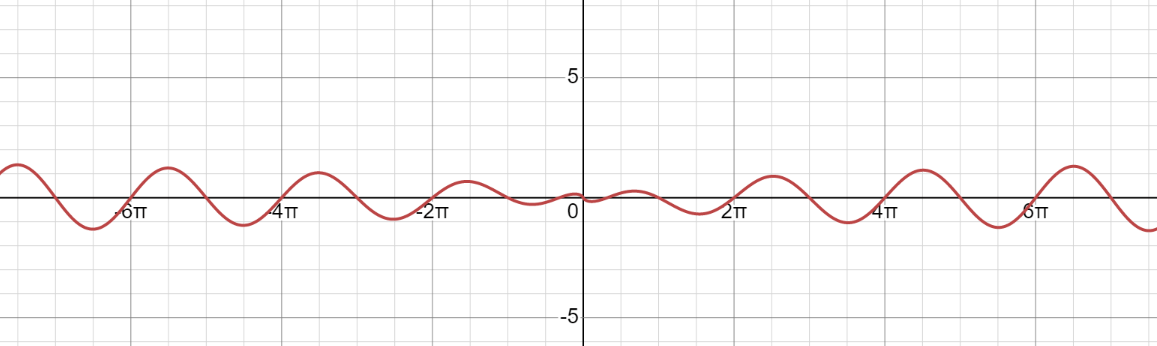×

Search anything:

# IISc Interview experience for CDS (Computational Data Science) PhD and M.Tech (Research)

#### Culture List of Interview QuestionsGet this book -> Problems on Array: For Interviews and Competitive Programming

In this article at OpenGenus, we have presented the IISc Interview experience for CDS (Computational Data Science) PhD and M.Tech (Research). We have added the actual questions asked in the Interview so that you can prepare well.

1. Phase 1: Written Test
2. Phase 2: Face to Face Interviews

### Phase 1: Written Test

Written Test Date: 2 May 2023 3:00 PM
Duration: 75 minutes

The written test had 12 MCQs and 2 Programming Questions.

• 4 Probability questions
• 3 Linear Algebra Questions
• 1 General Aptitude Question
• 2 Calculus Questions
• 2 Algorithmic Questions

Following are the 12 MCQs:

• Q1: A man forgets the last digit of a telephone number and dials the last digit at random. What is the probability of calling the correct number within 4 trails?

P(X=i) = Probability of getting the number correct in i-th trail so first i-1 trails are not correct.
P(X=1) = 1/10
P(X=2) = 9/10 * 1/9 = 1/10
P(X=3) = 9/10 * 8/9 * 1/8 = 1/10
P(X=4) = 9/10 * 8/9 * 7/8 * 1/7 = 1/10
Answer: P(X=1) + P(X=2) + P(X=3) + P(X=4) = 4/10 = 2/5

• Q2: There are 5 different types of unlimited t-shirts. How many different ways you can select 10 t-shirts?
Answer 2: 5^10 = 97,65,625 ways

• Q3: Suppose a computer program has an error rate of 4.5%. This means there is a 4.5% chance that the program will output an incorrect result. If the program is run 100 times on independent inputs, what is the probability that there will be at least one incorrect output?

The problem is solved using complement rule.

1 - (1-0.045)^100 = 98.99% chance

• Q4: Suppose a fair coin is flipped repeatedly until the first time a head appears. Let X be the number of flips required. What is the probability that X is odd?

• Q5: Let A be a matrix. Given trace(A) and A^3. Find the matrix A.

Answer 5: Trace is defined for square matrix only so A is a square matrix. trace(A) = sum of diagonal elements.

• Q6: Let M = A - A^T + AA^T (T is transpose). What is M? (symmetric/ skew symmetric / symmetric only if A is symmetric)

Answer 6: M is symmetric only if A is symmetric.
M^T = A^T - A + AA^T
If A is symmetric, then A^T = A, so M will be symmetric.

• Q7: Let A be a 2 x 2 matrix such that trace (A) = det (A) = 3. What is trace (A^-1)?

Answer 7: Let a and b be diagonal elements in A.
trace(A) = a + b = 3
DET(A) = a * b = 3
So, trace(inv(A)) = 1/a + 1/b = (a+b)/ab = 1

• Q8: The percent profit made when a pen is sold for Rs. 78 is twice as much as when it is sold for Rs. 69. Find the cost price of the pen?

• Q9: dy/dx=u d2y/dx2=v then d2x/dy2==?

• Q10: Plot function f(x) = sin(x) * log(|x|)• Q11: What is the worst case time complexity for searching in Binary Search Tree?
Answer 11: The worst case time complexity for searching in Binary Search Tree is O(N). This happens when BST is left or right skewed.

• Q12:

Following are the 2 Programming Questions:

Q1: Find the minimum number of edges that need to be removed from a graph in order to disconnect any two given vertices.

Q2: Graph is given as adjacency matrix. Standard All pair shortest path Floyd warshall algorithm. After calculating it find the largest of the calculated distances.

### Phase 2: Face to Face Interviews

Key points of the Face to Face Interview:

• Duration: ~ 1 hour
• Panel of 4 Professors

For my position, the professors focused on two domains:

• Data Science
• Operating System

There was a sheet of paper where we are allowed to take notes or demonstrate a program or proof as and when requested by the professors.

This is a stressful round. The professors will start with basic theoretical questions but will quickly, move to research type questions and want to see your thinking process.

Best of Luck.

#### Rahul Reddy

M.Tech in Computer Science and Engineering from IIT Bhubaneswar | SDE Intern at Flipkart

IISc Interview experience for CDS (Computational Data Science) PhD and M.Tech (Research)